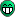# Need to improve fitting

Hello,

I have a distribution which is similar to gaussian but the tail of right side of it is not following the patteren. As shown in fit.pdf (14.4 KB)
Please tell me how can I improve my fitting. Thanks in advance.

Your distribution seems to be a bit asymmetric, so the simplest solution could be a sum of two symmetric distributions -> e.g. the Gaussian that you use now plus another smaller and broader distribution (not necessarily another Gaussian) shifted a bit to the left.

As symmetric distributions, you could try, for example:

1. the “Normal a.k.a. Gaussian distribution” -> TMath::Gaus
2. the “Cauchy a.k.a. Lorentz distribution” -> TMath::CauchyDist
3. the “Voigt profile” -> TMath::Voigt
4. the “Student’s t-distribution” -> TMath::Student
5. the “Q-Gaussian” (not in TMath nor TFormula)
6. the “Logistic distribution” (not in TMath nor TFormula)

Hello Coyote,

I was trying your suggestion. For that I thought lets first fit with the individual function then try the combined one. But the individual function is not working. Even when I define the Gauss function as a function and then try to fit it, is not working. But when I do histogram ->Fit(“Gaus”); it works perfectly. Below is the code that I am trying and the plot that I am getting is also attached. Please suggest what I am doing wrong.

``````TCanvas *c2 = new TCanvas("c2","RUN060 X Offset for Run 060",1);
c2->Clear();
TH1F *H2 = new TH1F("H2","RUN060 X Offset for g2 wrt g1 (Run 60)",20,-4,4);
T_CRC60->Draw("g2xcl.geoposX-g1xcl.geoposX>>H2");
H2->SetStats(111111);
//	TF1 *f1 = new TF1("f1", "TMath::CauchyDist(x, , )", -4, 4);
//	f1->SetParameters(0, 0.1);

//	TF1 *f2 = new TF1("f2", "TMath::Voigt(x,,,4)",-4,4);
//	f2->SetParameters(1,1);

TF1 *f3 = new TF1("f3","TMath::Gaus(x,,,0)",-4,4);
f3->SetParameters(0,0.7);

H2->Fit("gaus");
//	H2->Fit("f3");
H2->Draw();
//	f1->Draw("same");
//	f3->Draw();
c2->SaveAs("fit.pdf");``````

Plot using Fit(“Gaus”); fit.pdf (14.2 KB)

Plot using Fit(“defined gauss function”); fit.pdf (14 KB)

 * TMath::Gaus(x, , , 0)

BTW. You could also try to play with the “W” (set all weights to 1) or the “L” (use Loglikelihood method) fit options.

Thanks Coyote. It worked.

Then I added the gaussian and Cauchy distribution. And Now the plot is fitted perfectly. Here it is fit.pdf (14.3 KB)
But Now how could I get its mean and sigma?

[quote=“ramkrishna”]But Now how could I get its mean and sigma?[/quote] Calculate them from the fitted parameters of both partial distributions.Hello Coyote,

I was asking because the Cauchy function has no mean and variance defined. But as I studied then I come to know that I can take location parameter as mean then add the two means. But, Is there a way to get the sigma for Cauchy+gaussian?

I’ve got another idea … try: ```// a pseudo-gaussian with one "maximum" and two "sigmas" // (note: "maximum" != "mean" -> "mean" is shifted towards the bigger "sigma") TF1 *fg = new TF1("fg", " * TMath::Gaus(x, , ((x < ) ?  : ), 0)", -4.0, 4.0); fg->SetParameters(6000, 0, 0.9, 0.6);```

Thanks Coyote. I got idea form this and problem resolved.

But I would like to ask some more problem that I am facing. During fit we need to give parameter initial values (some guess that probably be the value of that parameter) when it does not work then we can try FixParameter or SetParLimits. But many times the fit is not looks ok. Then, we need to change the parameters and look the fitting again and again until the fit looks fine.

Is there any easier way instead of doing change parameter values again and again and look fit again and again. Because for example the histogram like Offset_Run060.pdf (14.6 KB) I tried many times but could not able to fit perfectly. Also I tried different functions sum of 2 Gaussian with different sigma, sum of a breit-wigner and Gaussian, etc.

Well, your histogram looks much like a “gaus”. So, try a two-step approach:

1. fit it with the default “gaus” -> ROOT should be able to find it automatically without problems
2. fit it with your own function, setting initial parameters using the fitted parameters from the step 1.

[code]{
gStyle->SetOptFit(1111); // print all available fit informations

// an asymmetric pseudo-gaussian with one “Position” and two “Sigmas”
// (“Position” != “Mean” -> “Mean” is shifted towards the bigger “Sigma”)
TF1 *f = new TF1(“f”, “ * TMath::Gaus(x, , ((x < ) ?  : ), 0)”, 0.0, 10.0);
f->SetParNames(“Height”, “Position”, “LeftSigma”, “RightSigma”);
f->SetParameters(1, 5, 1, 0.5);

// create the test histogram
TH1D *h = new TH1D(“h”, “asymmetric pseudo-gaussian”, 20, 0, 10);
h->FillRandom(“f”, 1000);

// fit with a simple "gaus"
h->Fit(“gaus”, “QWN”); // quiet, equal weights, do not store, do not draw
TF1 *fg = ((TF1 *)(gROOT->GetListOfFunctions()->FindObject(“gaus”)));
if (!fg) { std::cout << “Error: gaus NOT found!” << std::endl; }

// fit with the actual function (i.e. the asymmetric pseudo-gaussian)
if (fg) { // just a precaution
// fg->Print(); // just for fun
f->SetParameters( fg->GetParameter(0), // Height
fg->GetParameter(1), // Position
fg->GetParameter(2), // LeftSigma
fg->GetParameter(2) ); // RightSigma
}
h->Fit(f);
}[/code]

1 Like

Can you post your code here? I am having the same problem.